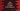# Java program to check if a number is a unique number or not## Java program to check if a number is a unique number or not:

In this Java programming tutorial, we will learn how to check if a number is unique or not. The program will take one number as input from the user, check if it is unique or not and print one message based on that.

### What is unique numbers:

A number is called unique if it is a positive number and no digit is repeated in the number. For example, 1234 is a unique number because it doesn’t have any repeated digit. But, 1231 is not. 1 is repeated.

We can check if a number is unique or not in different ways. In this post, I will show you three different ways to check for unique numbers in Java.

### Method 1: By comparing the rightmost digit with all other digits:

We can remove the last digit of the number and compare it with all other digits. The below program is comparing the rightmost digit to find if a number is unique or not:

``````import java.util.Scanner;

public class Main {
public static boolean digitExist(int n, int d) {
while (n > 0) {
if (n % 10 == d)
return true;
n = n / 10;
}
return false;
}

public static void main(String[] args) {
Scanner sc = new Scanner(System.in);
int number;
boolean isUnique = true;

System.out.println("Enter a number: ");
number = sc.nextInt();

while (number > 0) {
int lastDigit = number % 10;
number = number / 10;

if (digitExist(number, lastDigit)) {
isUnique = false;
break;
}
}

if (isUnique) {
System.out.println("It is a unique number");
} else {
System.out.println("It is not a unique number");
}
}
}``````

Here,

• sc is a Scanner object to read the user input.
• isUnique boolean is used to define if the number is unique or not. It is initialized as true. It is changed to false if the number is not unique.
• It is taking the number as input from the user and that value is stored in the variable number.
• The while loop will run until the value of number is greater than 0.

• It gets the last digit of the number and stores that in the lastDigit variable.
• It removes the last digit from the number by dividing it by 10.
• It calls digitExist method to check if the removed last digit is in the other part of the number or not. If yes, it assigns false to isUnique and breaks from the loop.
• digitExist method takes one number and one digit as its parameters. It checks if the digit is in the number or not. Based on that, it returns one boolean value.

If you run this program, it will print output as like below:

``````Enter a number:
121345
It is not a unique number

Enter a number:
123456
It is a unique number

Enter a number:
100
It is not a unique number``````

### Method 2: By using ArrayList:

We can also use an ArrayList. The program will keep removing the last digit from the number and check if it exists in the ArrayList or not. If it exists, it is not an unique number. Else, it will insert that into the ArrayList.

``````import java.util.ArrayList;
import java.util.Scanner;

public class Main {

public static void main(String[] args) {
Scanner sc = new Scanner(System.in);
ArrayList<Integer> arrayList = new ArrayList<>();
int number;
boolean isUnique = true;

System.out.println("Enter a number: ");
number = sc.nextInt();

while (number > 0) {
int lastDigit = number % 10;
number = number / 10;

if (arrayList.contains(lastDigit)) {
isUnique = false;
break;
}

}

if (isUnique) {
System.out.println("It is a unique number");
} else {
System.out.println("It is not a unique number");
}
}
}``````

It is almost similar to the above program.

• The while loop runs until the value of number is not zero.
• It removes the last digit of the number and checks if it is in the ArrayList or not by using the contains method.

• If it exists, it assigns false to isUnique and exists from the loop.
• Else, it adds that number to the arraylist.
• Based on the value of isUnique, it prints one message.

If you run this program, it will print similar results.

### Method 3: By using a HashMap:

We can also use a HashMap. HashMap is used to store key-value pairs. The program will remove the last digit of the number and add it as key to the HashMap with value as 0. If this key already exist, it will mark it as non-unique number.

``````import java.util.HashMap;
import java.util.Scanner;

public class Main {

public static void main(String[] args) {
Scanner sc = new Scanner(System.in);
HashMap<Integer, Integer> map = new HashMap<>();
int number;
boolean isUnique = true;

System.out.println("Enter a number: ");
number = sc.nextInt();

while (number > 0) {
int lastDigit = number % 10;
number = number / 10;

if (map.containsKey(lastDigit)) {
isUnique = false;
break;
}

map.put(lastDigit, 0);
}

if (isUnique) {
System.out.println("It is a unique number");
} else {
System.out.println("It is not a unique number");
}
}
}``````

Here,

• map is the HashMap that can hold key and values of Integer types.
• It uses containsKey method to check if the key exists in the HashMap or not.
• If it exists, it assigns false to isUnique and exists from the loop.
• Else, it uses the put method to insert the last digit as key and 0 as its value.

It will give similar output.